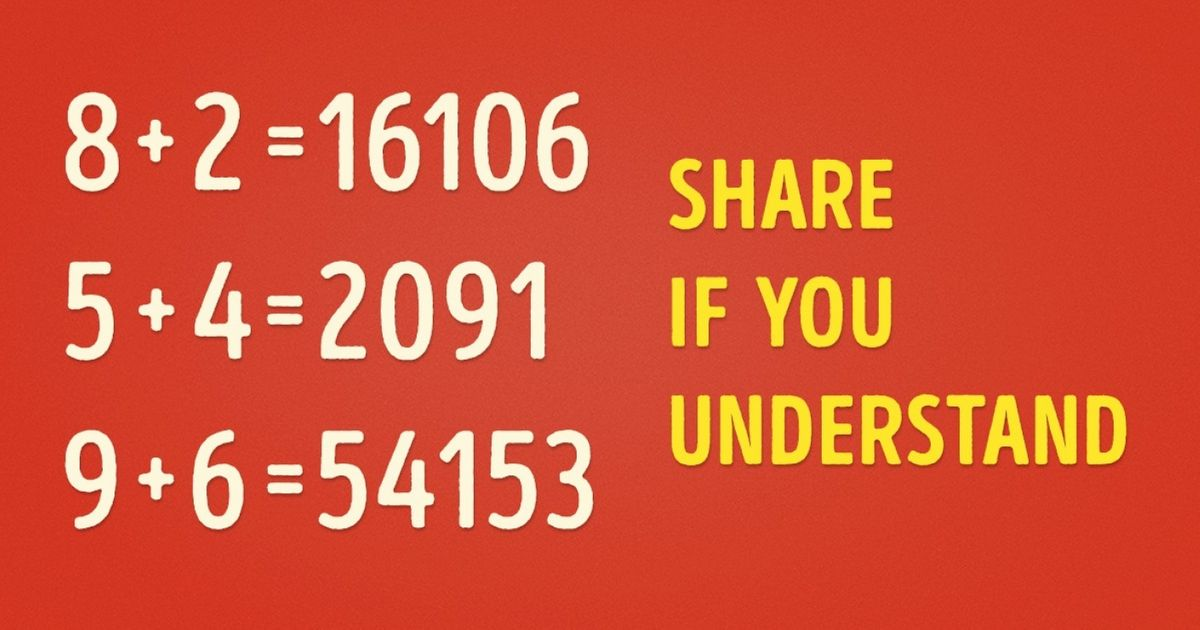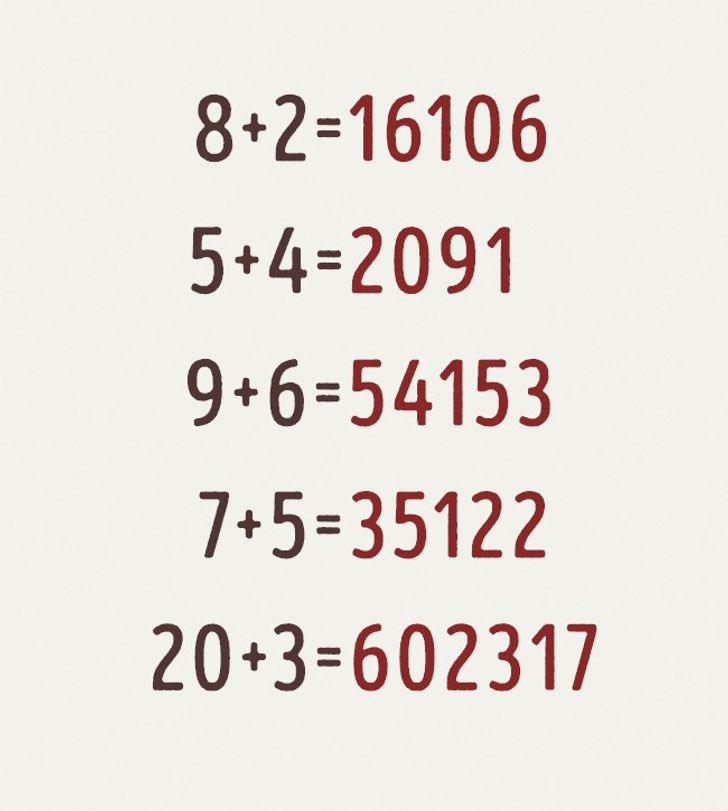# If You Can Solve This Math Equation, Then You Really Are A Genius

Try to figure out what these equations have in common:.

.

.

.

.

Every number following the equals sign reflects the outcomes of three sums generated by multiplying, adding, and subtracting the two numbers before the equals sign.

For example, 8 + 2 = 16106

8 x 2 = 16
8 + 2 = 10
8 – 2 = 6

The same concept applies to the remaining equations. Do you believe your buddies will be able to get it as quickly as you did now that you know?